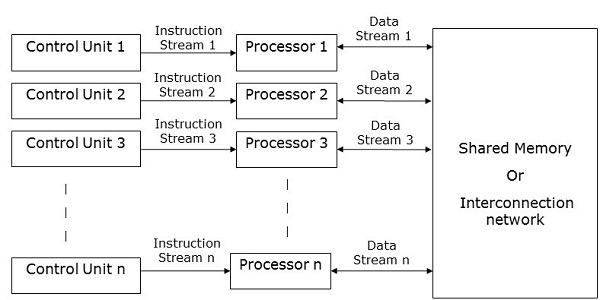# Parallel Algorithm - Introduction

An algorithm is a sequence of steps that take inputs from the user and after some computation, produces an output. A parallel algorithm is an algorithm that can execute several instructions simultaneously on different processing devices and then combine all the individual outputs to produce the final result.

## Concurrent Processing

The easy availability of computers along with the growth of Internet has changed the way we store and process data. We are living in a day and age where data is available in abundance. Every day we deal with huge volumes of data that require complex computing and that too, in quick time. Sometimes, we need to fetch data from similar or interrelated events that occur simultaneously. This is where we require concurrent processing that can divide a complex task and process it multiple systems to produce the output in quick time.

Concurrent processing is essential where the task involves processing a huge bulk of complex data. Examples include − accessing large databases, aircraft testing, astronomical calculations, atomic and nuclear physics, biomedical analysis, economic planning, image processing, robotics, weather forecasting, web-based services, etc.

## What is Parallelism?

Parallelism is the process of processing several set of instructions simultaneously. It reduces the total computational time. Parallelism can be implemented by using parallel computers, i.e. a computer with many processors. Parallel computers require parallel algorithm, programming languages, compilers and operating system that support multitasking.

In this tutorial, we will discuss only about parallel algorithms. Before moving further, let us first discuss about algorithms and their types.

## What is an Algorithm?

An algorithm is a sequence of instructions followed to solve a problem. While designing an algorithm, we should consider the architecture of computer on which the algorithm will be executed. As per the architecture, there are two types of computers −

• Sequential Computer
• Parallel Computer

Depending on the architecture of computers, we have two types of algorithms −

• Sequential Algorithm − An algorithm in which some consecutive steps of instructions are executed in a chronological order to solve a problem.

• Parallel Algorithm − The problem is divided into sub-problems and are executed in parallel to get individual outputs. Later on, these individual outputs are combined together to get the final desired output.

It is not easy to divide a large problem into sub-problems. Sub-problems may have data dependency among them. Therefore, the processors have to communicate with each other to solve the problem.

It has been found that the time needed by the processors in communicating with each other is more than the actual processing time. So, while designing a parallel algorithm, proper CPU utilization should be considered to get an efficient algorithm.

To design an algorithm properly, we must have a clear idea of the basic model of computation in a parallel computer.

## Model of Computation

Both sequential and parallel computers operate on a set (stream) of instructions called algorithms. These set of instructions (algorithm) instruct the computer about what it has to do in each step.

Depending on the instruction stream and data stream, computers can be classified into four categories −

• Single Instruction stream, Single Data stream (SISD) computers
• Single Instruction stream, Multiple Data stream (SIMD) computers
• Multiple Instruction stream, Single Data stream (MISD) computers
• Multiple Instruction stream, Multiple Data stream (MIMD) computers

## SISD Computers

SISD computers contain one control unit, one processing unit, and one memory unit.In this type of computers, the processor receives a single stream of instructions from the control unit and operates on a single stream of data from the memory unit. During computation, at each step, the processor receives one instruction from the control unit and operates on a single data received from the memory unit.

## SIMD Computers

SIMD computers contain one control unit, multiple processing units, and shared memory or interconnection network.Here, one single control unit sends instructions to all processing units. During computation, at each step, all the processors receive a single set of instructions from the control unit and operate on different set of data from the memory unit.

Each of the processing units has its own local memory unit to store both data and instructions. In SIMD computers, processors need to communicate among themselves. This is done by shared memory or by interconnection network.

While some of the processors execute a set of instructions, the remaining processors wait for their next set of instructions. Instructions from the control unit decides which processor will be active (execute instructions) or inactive (wait for next instruction).

## MISD Computers

As the name suggests, MISD computers contain multiple control units, multiple processing units, and one common memory unit.Here, each processor has its own control unit and they share a common memory unit. All the processors get instructions individually from their own control unit and they operate on a single stream of data as per the instructions they have received from their respective control units. This processor operates simultaneously.

## MIMD Computers

MIMD computers have multiple control units, multiple processing units, and a shared memory or interconnection network.Here, each processor has its own control unit, local memory unit, and arithmetic and logic unit. They receive different sets of instructions from their respective control units and operate on different sets of data.

### Note

• An MIMD computer that shares a common memory is known as multiprocessors, while those that uses an interconnection network is known as multicomputers.

• Based on the physical distance of the processors, multicomputers are of two types −

• Multicomputer − When all the processors are very close to one another (e.g., in the same room).

• Distributed system − When all the processors are far away from one another (e.g.- in the different cities)